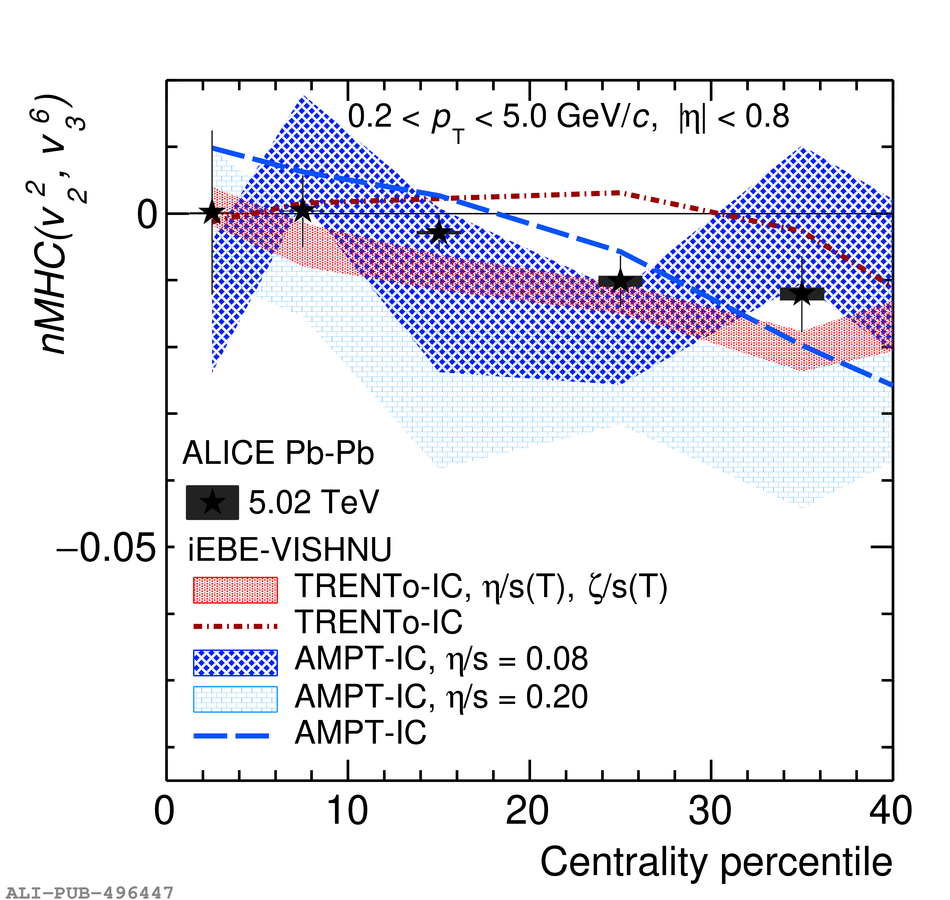# Figure 7

 Centrality dependence of $nMHC(v_{2}^{2}, v_{3}^{6})$ in \PbPb\ collisions at $\sqrt{s_{\rm NN}} = 5.02$ TeV. Statistical uncertainties are shown as vertical bars and systematic uncertainties as filled boxes.